# How to Use Lumped Elements to Model a Mechanical System

May 29, 2019

You can use the Lumped Mechanical System interface to develop 1D mechanical circuit models using lumped elements such as masses, springs, and dampers. Such circuit or lumped models can be easily coupled with standard finite element (FE) models, which is useful when simulating complex mechanical systems.

### Working with Lumped Mechanical Systems

In general, all of the components of a mechanical system can essentially be modeled as a combination of lumped mass, stiffness, and damping parameters. The finite element method (FEM) uses a similar analogy to distribute these parameters on mesh elements and assemble them to form complete mass, stiffness, and damping matrices for each component.

When modeling large complex systems, it is useful to simplify various configurations in the model setup in order to develop a better understanding of the system. For example, consider the vibration analysis of a vehicle due to unidirectional ground excitation. Using a simplified approach, the car tires can be modeled using a spring-damper system, ignoring the nonlinear behavior of the tires. Similarly, if you want to simulate the human body using a lumped approach, the Lumped Mechanical System interface can be used to compute the response of different parts of the body.

Other uses of the lumped approach include electroacoustics analyses, where the different physics (electrical, mechanical, etc.) can be modeled through lumped elements (such as resistors, inductors, capacitors, masses, springs, and dampers).

Let’s discuss how the equivalent circuits for various type of systems can be developed and solved.

#### The Mechanical and Electrical Analogy

The theory of mechanical circuits is derived from the concept of electrical circuits. Once the connection or analogy between mechanical and electrical circuits is established, the circuit theory used for pure electrical systems can be used to analyze mechanical systems. The methodology used is as follows:

1. Establish an analogy between an electric system and mechanical system
2. Solve the electrical system using the electric circuit theory
3. Convert the solution into mechanical quantities

These analogies are developed to find a relationship between identical mathematical variables used in mechanical and electrical physics. Numerous analogies are theoretically possible, but two analogies are widely used: impedance and mobility.

For a mechanical system, impedance is the ratio of force and velocity, whereas mobility is the ratio of velocity and force (=1/impedance). The impedance analogy makes the force and voltage analogous, whereas mobility makes velocity and current analogous. These kinds of analogies are very helpful when defining electromechanical systems, since such systems have a connection between electrical and mechanical parts.

Let’s discuss the electrical analogy in little more detail. Consider a mass-spring-damper system, where the spring acts as a storage element and is equivalent to a capacitor in an electrical circuit. The damper acts as a dissipative element and is equivalent to a resistor. The mass is an inertial element and equivalent to an inductance. This analogy with an electrical system is popularly known as the mobility analogy, in which the velocity computed from the mechanical system (by the application of force) is equivalent to the current obtained from the electrical system (by the application of voltage). The equivalent systems are shown in the figure below.

Left: A lumped mechanical system (impedance analogy). Right: An equivalent electrical circuit (mobility analogy).

Let’s consider an example of a loudspeaker driver system comprised of a mass-spring-damper system, where the diaphragm and voice coil form the mass, spider, and surround (as shown in the loudspeaker driver diagram below) constitutes to the spring element. The losses in the suspension can be modeled as a damping element. The equation of motion for such a system (such as the lumped and electrical circuit shown above) is given by:

\frac{F}{v}=R+\frac{1}{i \omega C}+i \omega L

where m=L, k=\frac{1}{C}, c=R, F=V, and v=i.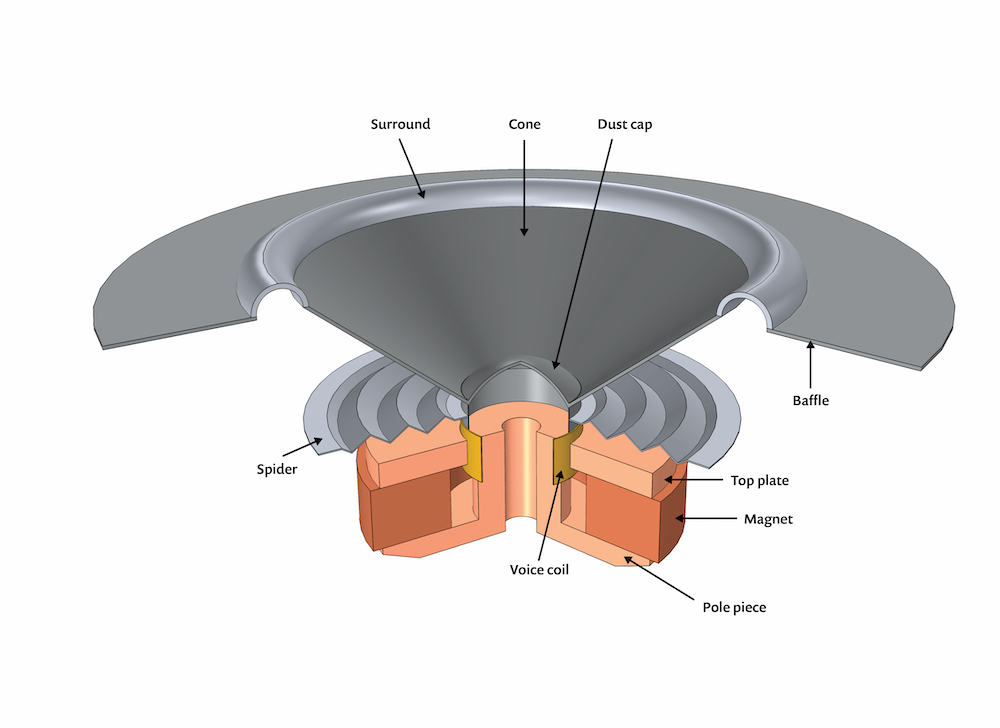Schematic representation of a typical loudspeaker driver.

This equation yields the mechanical impedance (\frac{F}{v}) model to the LRC circuit in series, which signifies that the LRC circuit in series is the same as the mechanical circuit in parallel. A parallel LRC circuit is analogous to a mechanical mobility model (when the mass, spring, and damper are arranged in series).

The mechanical and electrical analogy is discussed in greater detail in two Application Gallery examples: a tutorial model of a lumped loudspeaker driver and a corresponding tutorial with mechanical components included.

#### Physics Interfaces for Lumped Modeling

The Lumped Mechanical System interface, available as of version 5.4 of the COMSOL Multiphysics® software, provides different lumped components (such as masses and springs) that can be arranged in series or parallel to analyze individual displacements and forces in one dimension. The limitation of modeling in 1D can be overcome by coupling the lumped models with multibody models, which support 2D and 3D elements.

For example, consider a car model with six DOFs — three translations and three rotations — and an assumed excitation from the ground that is unidirectional. This excitation can be modeled using the lumped approach and coupled with the distributed model in order to account for the effect of all six DOFs. The distributed model can be built using either the Solid Mechanics interface or Multibody Dynamics interface. Another example is modeling the vibration isolation of structures, where the isolators can be modeled using a spring-damper system and attached to a mass with multiple DOFs.

Next, let’s discuss how to use this approach to develop mechanical circuit models in COMSOL Multiphysics.

### The Components of a Mechanical Circuit Model

We begin with a schematic representation of a lumped system, marking the ports across each component (such as the mass, spring, and damper). Then, we apply excitation and solve the problem for displacements and forces.

The components in a mechanical circuit are divided into two-port components and single-port components. Considering the two-port components, displacements and forces are applied across the components (such as the mass, spring, damper, and impedance). For example, displacements across mass are the same, whereas there is a differential in terms of force. Similarly, forces across the spring element are the same, with a differential in terms of displacement. The generalized equations for a two-port network (as shown below, where p_1 and p_2 represent the ports across the component) are given by: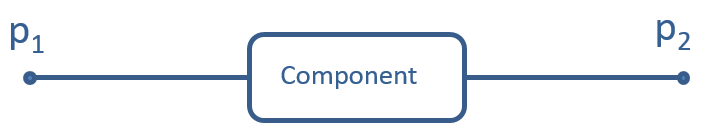f_{p_1}+f_{p_2}=f
u_{p_2}-u_{p_1}=u
f_{p_2}=f_p
u_{p_2}=u_p

where f_{p_1}, f_{p_2} and u_{p_1}, u_{p_2} represent the forces and displacements at ports p_1 and p_2, respectively.

The two-port components available in the Lumped Mechanical System interface in COMSOL Multiphysics are listed in the table below:

Two-Port Component Schematic Representation
f

u

f_p

u_p
Mass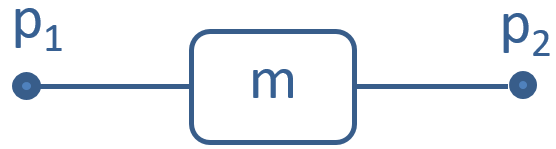m \ddot{u_p}

0

0

u_p
Spring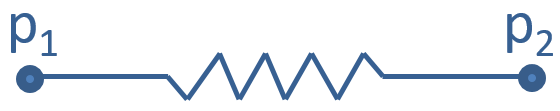0

u

-ku

0
Damper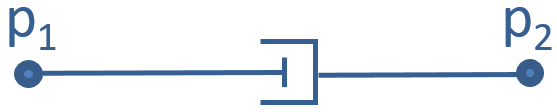0

u

-c \dot{u}

0
Impedance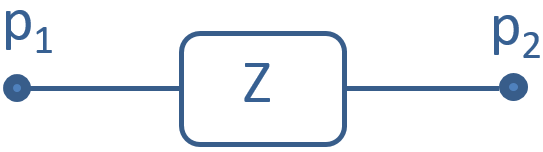\begin{bmatrix}
f_{p_1}\\
f_{p_2}
\end{bmatrix}=\begin{bmatrix}
Z_{11} & Z_{12}\\
Z_{21} & Z_{22}
\end{bmatrix} \begin{bmatrix}
\dot u_{p_1}\\
\dot u_{p_2}
\end{bmatrix}
Displacement source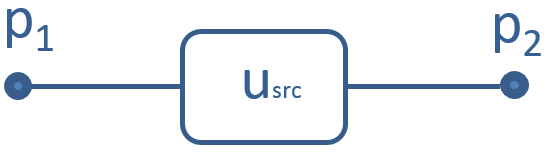0

u

f
Force source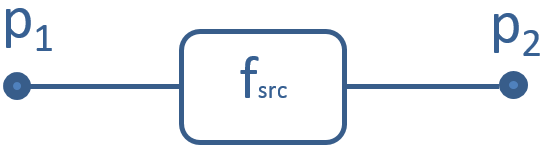f

0

u
External source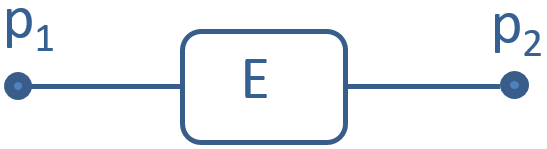f_{p_1}=f_1, u_{p_1}=u_1,f_{p_2}=f_2, u_{p_2}=u_2

In the table above, m is the mass; k is the stiffness of the spring; c is the damping coefficient; and \dot u and \ddot u represent the velocity and acceleration of the component, respectively.

Along with the standard components, several other components are introduced in this interface. One of them is the impedance, which is used to create a difference in both displacements and forces. Another one is the external source, which can be used to connect a distributed model to the lumped model of a system. An external source connects two nodes in a mechanical system. The equations for the external source can be defined within or outside of the Lumped Mechanical System interface. The displacement of both the nodes from the distributed model can be specified as input to the lumped model using the external source.

In the screenshot below, the point displacement is used as an input for ports 2 and 4, respectively. Correspondingly, the forces computed at this port are transferred to the Multibody Dynamics interface as inputs. This ensures the continuity of displacements and forces at those points.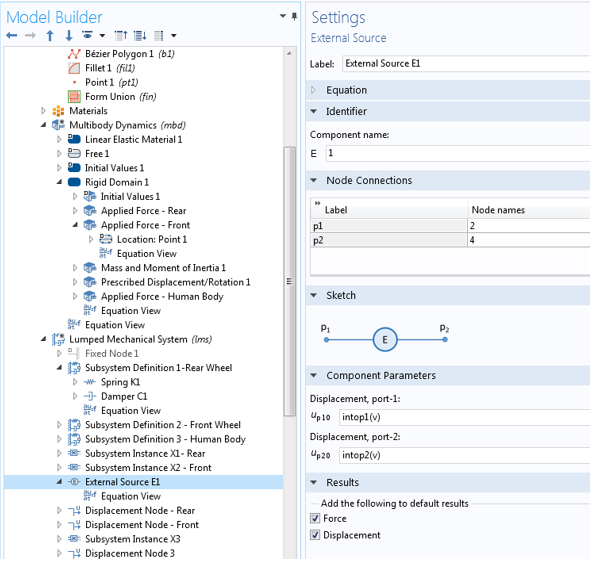Coupling of displacements and forces between distributed and lumped models.

Subsystem Definition nodes are used to define subsystems. Under this node, all of the components are accessible to define the subsystem, which can then be inserted as a component in the main system using the Subsystem Instance node. For example, consider a car model that is comprised of four identical tires and five seats. Suppose the tires and seat are modeled using a spring-damper system that independently uses a different configuration. Instead of creating component models with four tires and five seats, a subsystem can be created for one tire and one seat. A Subsystem Instance node is used to define the instance of each subsystem, which can then be used to connect the subsystem with the other system.

Single-port components are used to assign boundary conditions in the form of displacement, force, and impedance on a single port of an n-port network; for instance, to model mechanical circuits and coupling with distributed models at a single point. The single-port components or terminals available in the Lumped Mechanical System interface in COMSOL Multiphysics are listed in the following table:

Single-Port Components Schematic Representation Description
Fixed node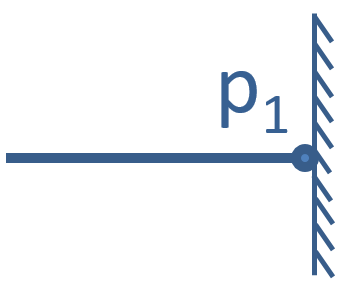u_{p_1}=0
Displacement nodeu_{p_1}=u
Velocity node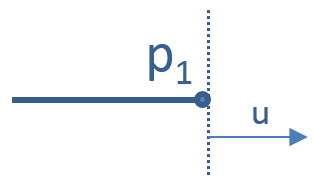v_{p_1}=\dot u=\frac{\partial u}{\partial t}
Acceleration node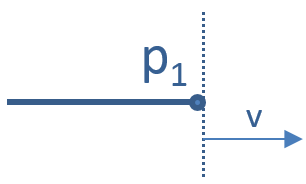a_{p_1}=\ddot u = \frac{\partial^2 u}{\partial t^2}
Free node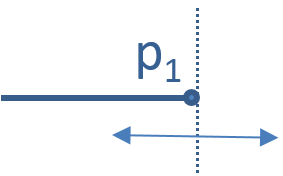f_{p_1}=0
Force node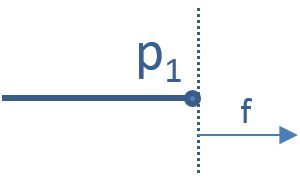f_{p_1}=f
Impedance node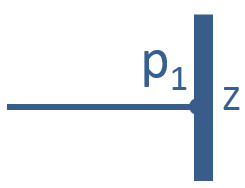Z=\frac{f_{p_1}}{v_{p_1}}

### Implementing a Lumped Mechanical Circuit in COMSOL Multiphysics®

To model a mechanical circuit in the COMSOL® software, the first step is to create the circuit and label all of its ports. For example, if a mass, spring, and damper are connected in parallel, the circuit will be comprised of three ports (1, 2, and 3). Mass can be assigned between ports 1 and 2, and the spring and damper can be located between ports 2 and 3 (see the image below).

This circuit can either be used as a subsystem or appropriate boundary/terminal conditions can be applied to get the displacement/forces on the components. Consider that the system is excited harmonically with a unit force at port 1, whereas port 3 is considered fixed. These port conditions can be assigned using a Force node and Fixed node, respectively, and a harmonic response can be computed using a frequency-domain study.

The values used in the numerical experiment are:

• Mass, m = 10 kg
• Stiffness, k = 1e4 N/m
• Damping coefficient, c = 10 N*s/m

The frequency response of the mass-spring-damper system is computed for a frequency range of 1 to 10 Hz with a frequency step of 0.1 Hz. The image below shows the amplitude of the displacement u vs. frequency.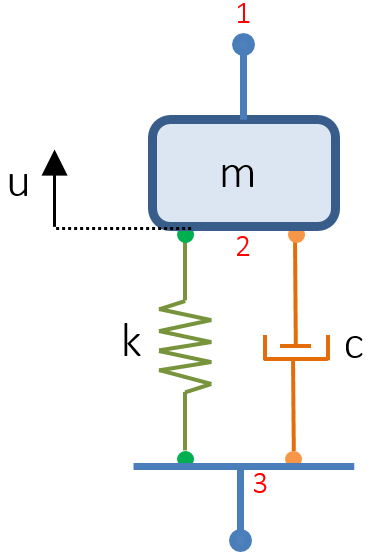A schematic of a mass-spring-damper system represented using a two-port component.The computed frequency response.

If a complex network of these elements is to be connected, then all of the nodes should be assigned a number. Once the numbers are assigned, appropriate elements are selected in COMSOL Multiphysics and the node numbers are prescribed along with the properties of the elements, such as stiffness for springs, damping coefficients for dampers, and so on.

If there are a number of subsystems present in a main system, then these subsystems can be modeled using the Subsystem Definition node in the Lumped Mechanical System interface. We’ll use a model of a human body created using lumped elements to demonstrate. This four-body model, also called an LN model, is one of the most commonly used lumped representations of a human body. The model consists of four masses (m_1,m_2,m_3,m_4); five springs (k_1, k_2, k_3, k_4, k_5); and three dampers (c_1, c_2, c_3).

In the model, the entire human body is divided into four parts:

1. Lower rigid mass (m_1)
2. Lower wobbling mass (m_2)
3. Upper rigid mass (m_3)
4. Upper wobbling mass (m_4)

Here, the wobbling mass includes the mass of all nonrigid parts, such as muscles, skin, and blood vessels. As seen below, the body model is comprised of 4 DOFs with the components labeled from 0 to a. The system has a terminal (labeled a), which can be used for excitation. A model of this type can be used to compute the response on any mass of the system for different excitations. Suppose we need to compute whole body vibration (WBV) when a person is sitting in a car. Terminal a can be attached to any point in the car model (distributed model) and the displacement of the car at that point can be used as input to excite the lumped system.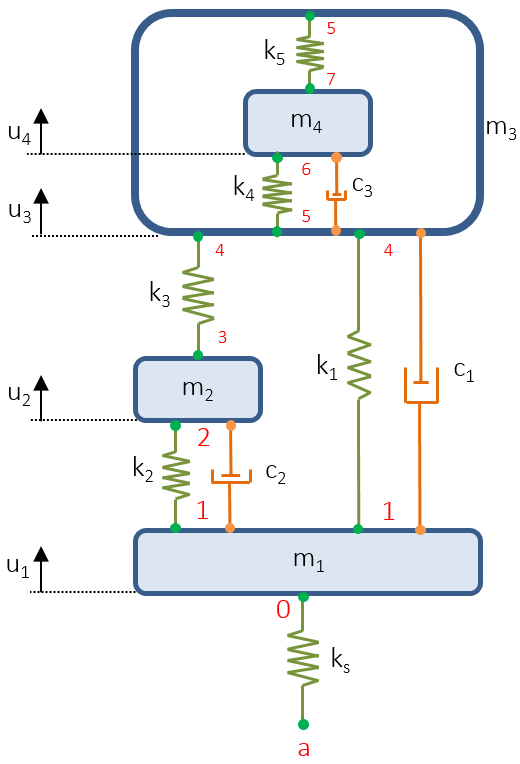Lumped model of a human body.

### Attaching a Mechanical Circuit Model to a Distributed System

As discussed in the previous section, in order to attach the lumped model to a distributed system (which can be a multibody system), the forces and displacements need to be coupled. Since the mechanical circuit is connected to the distributed system at a point, the displacements from the system are used as input to the mechanical circuit, whereas the forces are transferred from the circuit to the main system at the point of attachment (shown below).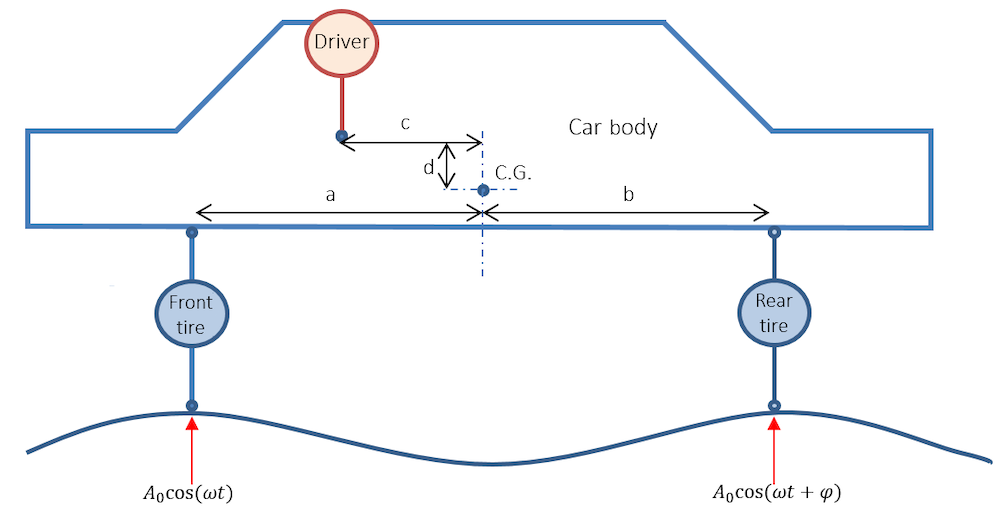Schematic representation of a car modeled using multibody dynamics, with the driver, front tires, and rear tires modeled using a lumped circuit.

In the example, a 2D car body is modeled using multibody dynamics. The car is comprised of front and rear tires with a driver sitting in the driver’s seat. The car is modeled using a rigid domain with a mass of m= 2667.24 kg and moment of inertia of 2788.07 kg/m2.

The tires get a harmonic excitation from the ground with a phase difference of 160 deg replicating bumps and undulations in the road. The tires are modeled using a spring and damper system attached in parallel. The stiffness and damping ratio of the tires are k_f= 553.28 kN/m, k_r= 496.38 kN/m, c_f= 2.374 kN/m/s, and c_r= 4.434 kN/m/s, respectively.

The human body’s mechanical circuit is used to model the driver. The details of the parameters used in the model are shown in the table below:

Parameter Value Unit

m_1
6.15 kg

m_2
6

m_3

12.58

m_4
50.34

k_1
6 kN/m

k_2
6

k_3
10

k_4
10

k_5
18

k_s
25.5

c_1
0.3 kN/m/s

c_2
0.65

c_3
1.9

The tires and the human body are connected to the car body at different points (as shown below).

Tires defined as a two-port subsystem (left) and the human body defined as a single-port subsystem (right).

The tires and the human body are defined using two-port and single-port subsystems, respectively. The advantage of using a subsystem is that, irrespective of the complexity of the model, it can be attached using one or two ports. Subsystem Instance nodes are then used to represent the instance of the subsystem.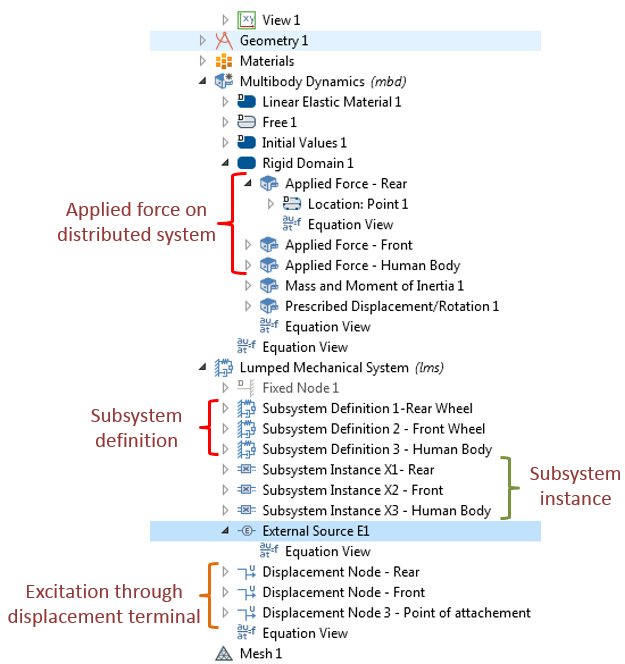Representation of the nodes in the lumped mechanical system and the distributed model (the Multibody Dynamics interface).

Again, the complete system is created by node numbering (as done while modeling the mechanical circuit for the human body). Three different Subsystem Instance nodes are used to represent the instance of the three different subsystems (namely the rear wheel, front wheel, and human body, respectively).

The External source option is selected to input displacements from the distributed model using the two-port system. Since the human body subsystem is a single-port subsystem, the displacements are prescribed using the Displacement Terminal node and the forces from this node are transferred to the distributed system at the point of attachment.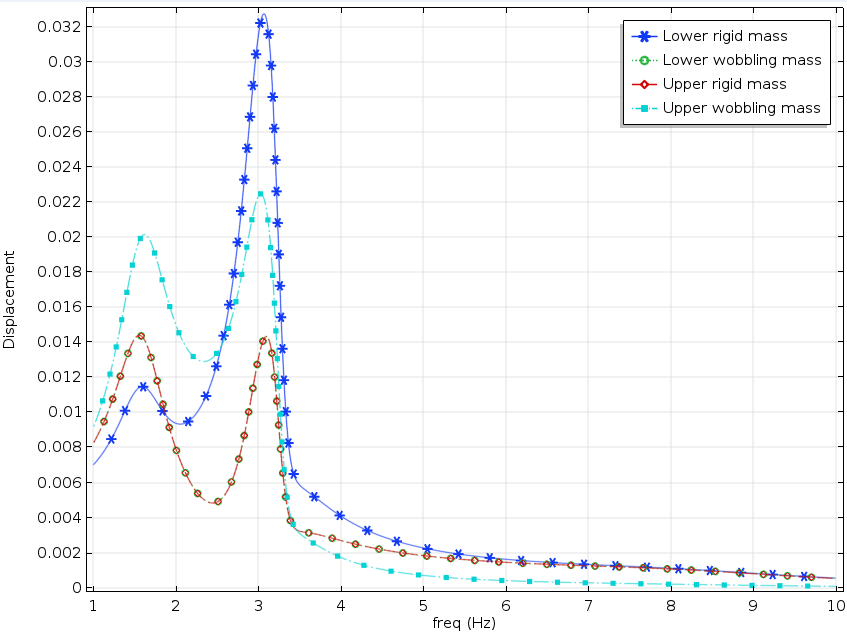Frequency response plot at the various components of the human body.

A frequency-domain analysis is performed for a frequency range of 1 to 10 Hz at a frequency step of 0.01 Hz. The frequency responses of various components are computed and plotted in the graph above. As shown, the response has a peak at 1.58 Hz, which is also the natural frequency of the human head.

In this blog post, we discuss the Lumped Mechanical System interface and explain its different components and applications, using example models of a mass-spring-damper and a human body lumped mechanical circuit. The procedure to couple a lumped circuit to a distributed system is demonstrated.

The Lumped Mechanical System interface proves to be a useful modeling tool. However, the limitations of using mechanical circuits is that they are confined to a single direction and handle only linear problems.

### Next Steps

Learn about the wide range of features available for structural analyses in the Structural Mechanics Module, an add-on to COMSOL Multiphysics, by clicking the button below. (Note that the functionality discussed here also requires the Multibody Dynamics Module.)